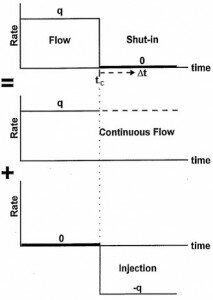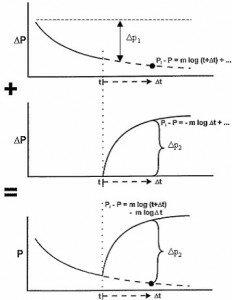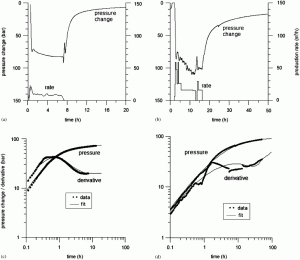# Superposition time in Well Testing

All derivation and results above were presented assuming constant rate production. In practice we need to model more complex flow histories. In particular, due to the difficulty of maintaining a constant flow rate. Pressure Transient Analysis is traditionally based upon build up data, preceded by one or more flowing and shut-in phases. It’s useful to apply a Superposition time in Well Testing .

Going from a single constant rate response to the simulation of complex production histories is possible using the principle of superposition in time, which also defines a superposition time functions to qualify flow regimes even for complex production histories.

THE GENERAL PRINCIPLE OF SUPERPOSITION

Superposition time in Well Testing and/or in space, consists in generating the solution of a complex problem with thel linear combination and superposition, in time and/or space, of simpler solutions. The most popular superposition in Pressure Transient Analysis and Production Analysis are:

1. Simulation of complex production historiesby linear combinations ofsimple drawdown solutions with different weights and starting times. This is called superposition in time.

2. Simulation of simple linear boundaries by linear combination of infinite well and interference solutions coming from virtual wells, simulating the boundary by symmetry (also called the method of image wells).

For any problem involving  linear diffusion equation, the main superposition principles are:

• Linear combinations of solutions honoring the diffusion equation also honor the equation.
• At any flux point (well, boundary), the flux resulting from the linear combination of solutions will be the same linear combination of the corresponding fluxes.
• If you find, from the linear combination of solutions that honor the diffusion equation, a solution that honors all flux and boundary conditions at any time, then it is THE solution of the problem. It does not matter that the elementary superposed solutions are physical or not, as long as the final combination honors the flux and boundary conditions.

From these principles it is easy to build the following series of rules for superposition in time:

• The pressure changes due to the production, Q, of a given system is Q times the unit rate solution of the same system. The solution for the injection at rate Q will be minus Q times the same unit rate solution.
• To simulate the sequence of a constant rate Q1 from time zero to time t1,FLOW ANALYSIS

followed by the production Q2 from time t1 ti infinity, you can superpose the porduction at rate Q1 from time zero to infinity and a production of rate (Q2-Q1) from time t1 to infinity.

• As a specific case, stimulate constant production Q from time o to production time tp, followed by a shut-in of infinite duration, you can superpose the production of Q from time zero to infinity and an injection of Q from time tp to infinity.
• This is easily extends to production sequences that may be as complex as needed.

## Build-up superposition time in Well Testing

The superposition time function has been  used as a tool to analyze transient pressure data measured under the influence of  a variable flow rate. This function is  usually defined assuming that radial flow  equations are valid, however, in practice  there are cases that exhibit several flow  regimes (i.e. fractured wells, partially  penetrating wells, etc.).SUPERPOSITION IN TIME BUILD UP TEST